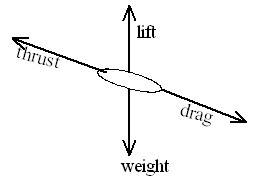# Velocity to forces (Newtons)

emperror123
1. an aeroplane of mass 300 tonnes during ascent travels at a constant velocity of 100 m/s. its angle of ascent is 10° to the horizontal. the trust produced by engines is 9000kN. Determine
i)the lift force acting on the wings
ii) the resistance to motion

i were trying to use f=ma, but the question didnt mention about the time, is it we need to convert 9000kN to acceleration in order to get time is second or can i get it from the question above, in other way i research via google with other website, some get F = 0N, is it possible?

secondly, the resistance is it = lift force or is it a drag force

thank

Homework Helper
Welcome to PF, emperror.
The plane is not accelerating, so both the vertical and horizontal forces must be zero. For the vertical forces, you have a component of thrust upward, lift upward and the weight downward. You also have a component of the drag (resistance to motion) downward. Looks like two unknowns there, so you will have to write a similar equation for the horizontal forces and solve the two as a system.

emperror123
u mean i have to find the vertical axis 1st before i can do the horizontal axis?
and i can assume that the velocity with angle with 0N?

thrust is it on horizontal or vertical? cause u said that the thrust is upward (y-axis), make me doubt on whether is it horizontal or vertical